## Absolute Stability for Quantized System

This example shows how to enforce absolute stability when a linear time-invariant system is in feedback interconnection with a static nonlinearity that belongs to a conic sector.

### Feedback Connection

Consider the feedback connection as shown in Figure 1.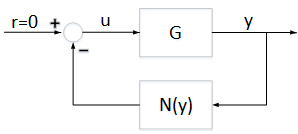Figure 1: Feedback connectionis a linear time invariant system, andis a static nonlinearity that belongs to a conic sector(where); that is,

``

For this example,is the following discrete-time system.

```addpath(fullfile(matlabroot,'examples','control','main')) % add example data A = [0.9995, 0.0100, 0.0001; -0.0020, 0.9995, 0.0106; 0, 0, 0.9978]; B = [0, 0.002, 0.04]'; C = [2.3948, 0.3303, 2.2726]; D = 0; G = ss(A,B,C,D,0.01); ```

### Sector Bounded Nonlinearity

In this example, the nonlinearityis the logarithmic quantizer, which is defined as follows:

``

where,. This quantizer belongs to a sector bound. For example, if, then the quantizer belongs to the conic sector [0.1818,1.8182].

```% Quantizer parameter rho = 0.1; % Lower bound alpha = 2*rho/(1+rho) % Upper bound beta = 2/(1+rho) ```
```alpha = 0.1818 beta = 1.8182 ```

Plot the sector bounds for the quantizer.

```PlotSectorBound(rho) ```represents the quantization density, where. Ifis larger, then the quantized value is more accurate. For more details about this quantizer, see .

### Conic Sector Condition for Absolute Stability

The conic sector matrix for the quantizer is given by

``

To guarantee stability of the feedback connection in Figure 1, the linear systemneeds to satisfy

``

where,andare the input and output of, respectively.

This condition can be verified by checking if the sector index,, is less than `1`.

Define the conic sector matrix for a quantizer with.

```Q = [1,-(alpha+beta)/2;-(alpha+beta)/2,alpha*beta]; ```

Get the sector index for `Q` and `G`.

```R = getSectorIndex([1;-G],-Q) ```
```R = 1.8247 ```

Since, the closed-loop system is not stable. To see this instability, use the following Simulink model.

```mdl = 'DTQuantization'; open_system(mdl) ```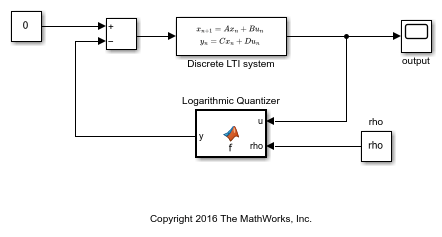```sim(mdl) open_system('DTQuantization/output') ```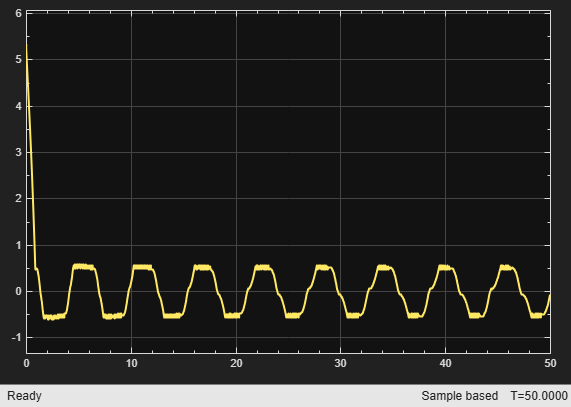From the output trajectory, it can be seen that the closed-loop system is not stable. This is because the quantizer withis too coarse.

Increase the quantization density by letting. The quantizer belongs to the conic sector [0.4,1.6].

```% Quantizer parameter rho = 0.25; % Lower bound alpha = 2*rho/(1+rho) % Upper bound beta = 2/(1+rho) ```
```alpha = 0.4000 beta = 1.6000 ```

Plot the sector bounds for the quantizer.

```PlotSectorBound(rho) ```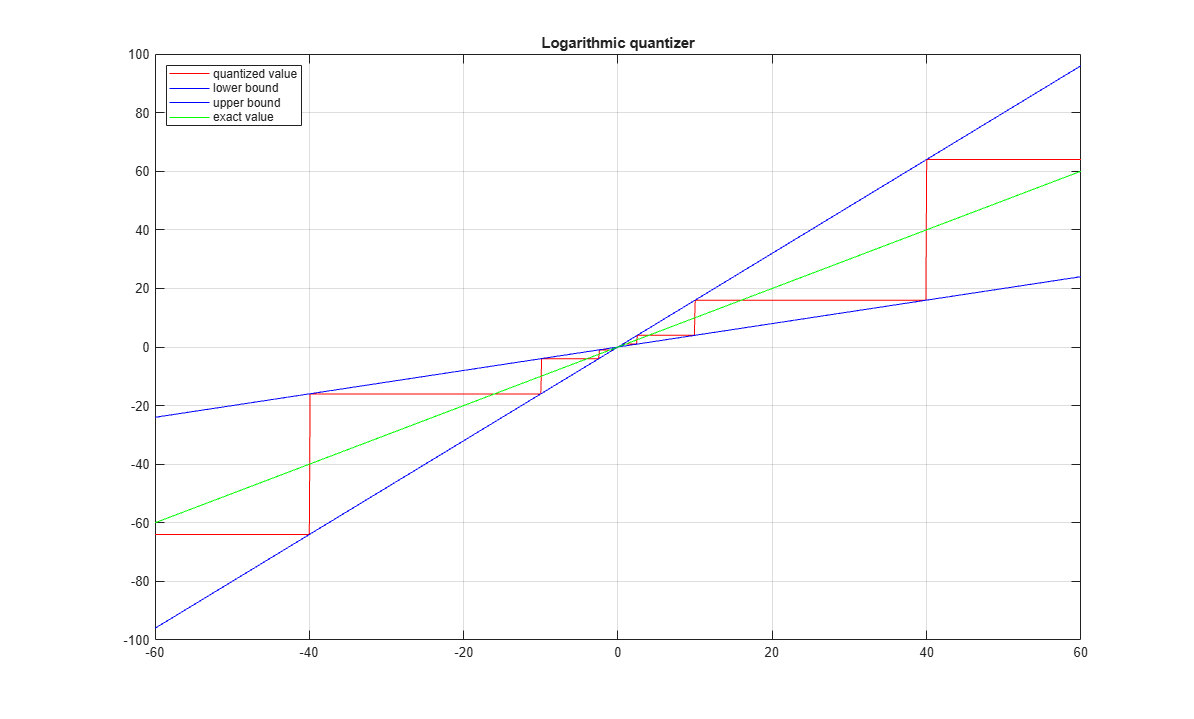Define the conic sector matrix for a quantizer with.

```Q = [1,-(alpha+beta)/2;-(alpha+beta)/2,alpha*beta]; ```

Get the sector index for `Q` and `G`.

```R = getSectorIndex([1;-G],-Q) ```
```R = 0.9702 ```

The quantizer withsatisfies the conic sector condition for stability of the feedback connection since.

Run the Simulink model with.

```sim(mdl) open_system('DTQuantization/output') ```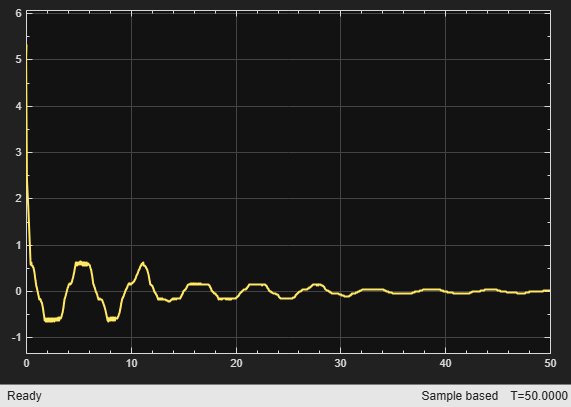As indicated by the sector index, the closed-loop system is stable.

### Reference

 M. Fu and L. Xie,"The sector bound approach to quantized feedback control," IEEE Transactions on Automatic Control 50(11), 2005, 1698-1711.

```bdclose(mdl); rmpath(fullfile(matlabroot,'examples','control','main')) % remove example data ```